# Operations on Relations

Besides the ordinary set operations, there are special operations we can perform on relations.

Definition. The inverse of a relation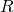from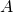to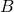is the relation fromtodefined by:That is,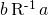iff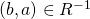iff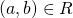iff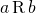.

E.g.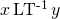iff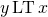iff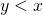.

That is, the inverse of the less-than relation is the greater-than relation.

Note that the inverse and the complement are very different things, because the complement of less-than is greater-than-or-equal-to.

Exercise. What is the inverse of the identity relation?

Definition. Ifis a relation fromtoand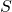is a relation fromto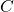, we define the {\em composite relation}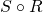fromtoby: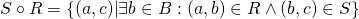Note that the source ofis the source ofand the target ofis the target of.

We can think of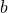as a stepping stone between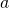and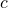.consists of all the pairs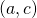such that there is such a stepping stone, as we pass from the source ofto the target of.

Observe that unless the target of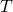and the source of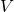are the same, we can't even make sense of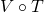. So most composites aren't defined, and we must first check that two relations are compatible before we try to compose them. Even ifis defined,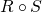usually isn't.

In lower mathematics courses, you probably only encountered relations on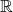, so that all sources and targets were the same---thus, all compositions were defined. The sole exception to this is in multivariable calculus. This is precisely why the multivariable Chain Rule is so much more complicated than its single-variable counterpart.

Exercise. Formulate the definition ofin infix notation. That is, explain what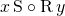means.

Exercise.  Consider the set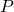of all people, and the relations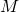andongiven by: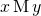iff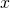is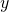's mother and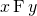iffis's father. Explain what the following relations mean: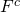,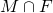,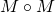,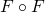,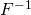,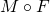,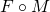,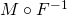,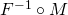.

Theorem. Letbe a relation fromto,a relation fromto, anda relation fromto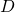. Then

•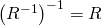•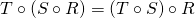•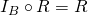and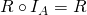•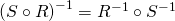•and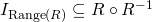.

Proof. We'll prove the last clause, and leave the others as exercises.

Since we need to prove, let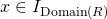. Then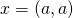for some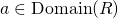. Since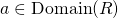, there is some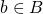with. So. So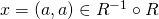.

To show, let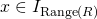. Then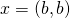for some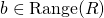. Since, there is some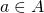with. Then. So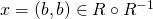.